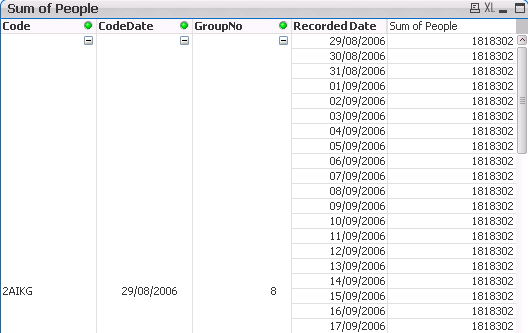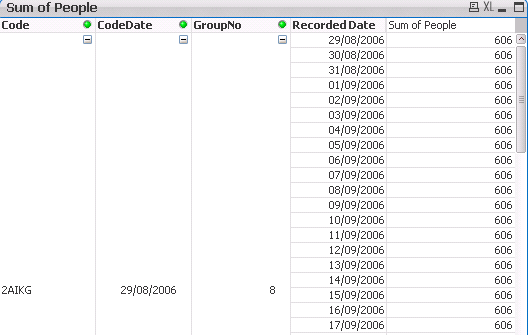QlikView Scripting

Discussion Board for collaboration on QlikView Scripting.

Highlighted
Contributor

First Row in Chart Expression returns table value and not Sum

Hi All,

I am trying to show the sum of people in a chart against every date which the key was recorded against in the database.

The Key is Code - CodeDate - GroupNo (2AIKG|29/08/2006|8)

It is working fine except for two rows in the chart.  The first row and a row in the middle.

The chart looks like this.As you can see the first line in the chart shows 3000 for recorded Date 29/08/2006 however I need to it show 12002 for that date.

Below is the data to show what the original looks like in a regular table.I did make another chart adding in the rank number to the chart for an explanation.So from what I understand the sum expressions 'Sum(People)' is summing on Code, CodeDate, GroupNo and Rank however I only want it to sum on Code, CodeDate and GroupNo. (Basically sum the middle table of this post for every recorded date).

Any ideas?

Tags (3)
1 Solution

Accepted SolutionsMVP

Re: First Row in Chart Expression returns table value and not Sum

Try this:

Sum(TOTAL <Code, CodeDate, GroupNo> Aggr(Test_One.people, Code, CodeDate, GroupNo, Test_One.Rank))

8 RepliesMVP

Re: First Row in Chart Expression returns table value and not Sum

What is the expression that you used? Is this what you are using?

Sum(TOTAL <Code, CodeDate, GroupNo> People)

Contributor

Re: First Row in Chart Expression returns table value and not Sum

The first table in the post above is simply sum(people).

I have tried sum(aggr(SUM(DISTINCT people), Code, CodeDate, GroupNo, Rank)) however it only returns a single row as shown below.MVP

Re: First Row in Chart Expression returns table value and not Sum

Can you try this:

Sum(TOTAL <Code, CodeDate, GroupNo> People)

or this

Aggr(NoDistinct Count(People), Code, CodeDate, GroupNo)

Contributor

Re: First Row in Chart Expression returns table value and not Sum

This expression -

Sum(TOTAL <Code, CodeDate, GroupNo> People)

Produces this:Which I believe is 12002 (Table 2 in original post) * Number of rows for Recorded Date.

And this expression -

Aggr(NoDistinct Count(People), Code, CodeDate, GroupNo)

Produces this:MVP

Re: First Row in Chart Expression returns table value and not Sum

Sorry, I meant

Aggr(NoDistinct Sum(People), Code, CodeDate, GroupNo)

But the above won't work as well... Will it possible for you to share a sample to look at the issue?

Contributor

Re: First Row in Chart Expression returns table value and not Sum

Yes of course.

Attached.

The reason I have written the script like it is, is because I want to create accumulative fields later on.

Thanks,MVP

Re: First Row in Chart Expression returns table value and not Sum

Try this:

Sum(TOTAL <Code, CodeDate, GroupNo> Aggr(Test_One.people, Code, CodeDate, GroupNo, Test_One.Rank))

Contributor

Re: First Row in Chart Expression returns table value and not Sum

That did the trick.

Thank you!